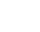# How to Make Sense of Functions on the ACT Math Test

Function questions are among the toughest problems you might see on the ACT Math Test. These questions sometimes ask about domain and range, or present you with a linear or quadratic function and ask you to plug in a number for a variable and solve. Functions can also use made-up symbol.

For these tricky problems, the test maker chooses a completely random symbol, something that you probably haven’t seen before (or at least not in a mathematical context), and give it a new definition.

You aren’t supposed to already know the meaning of the new symbol in the same way you know common math symbols like +, -, x and ÷. The question will tell you exactly how the new symbol functions. While these problems may seem intimidating, simple plugging in is usually all that is called for to get the right answer. Let’s try an easy one!

Let a € b be defined by the equation a € b = a2 – 1/b. What is the value of 3 € 1?

(A) 1
(B) 3
(C) 4
(D) 6
(E) 8

Here all we have to do is plug in 3 for a and 1 for b to solve.9 – 1 = 8, so the correct answer is (E).

This same kind of “monkey see, monkey do” logic can also be applied to non-symbol function questions. Let’s try one out:

If f(x) = 3x – 4 for all real numbers x, what is the value of the function f(x) when four times the value of  f(1/3) is plugged into the input f(x)?

(F) 50

(G) 40

(H) 30

(J) -40

(K) -30

We can translate this wordy question to essentially state: what is f(4(-3))? To solve this one, always start with the innermost parentheses. Since 1/3 is inside of 4, we’ll start by plugging in x = 1/3 into the function. That gives us f(x) = -3. Our question now looks like: what is f(4(-3))? The product of 4 and -3 is -12, so the question now becomes, what is f(-12). We have to plug in -12 into our function again:

f(x) = 3(-12) -4 = -36 – 4 = -40. The correct answer is (J).

For function questions, you can also look out for opportunities to use the answer choices to your advantage on symbol function questions! Plugging in and working backwards is one ACT Math strategy that really pays off, and the answer choices are a great place to look if you’re feeling stuck!

## Author

•Magoosh is a company of passionate educators, located in Berkeley, CA. We are here to provide you with everything you need to prep for the ACT, SAT, GRE, GMAT, and TOEFL! Be sure to follow us on Twitter @Magoosh.